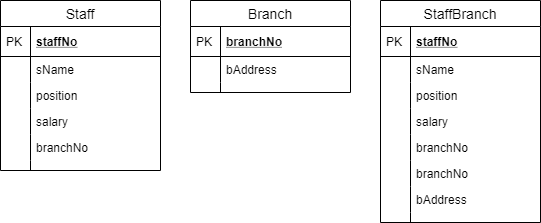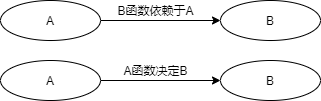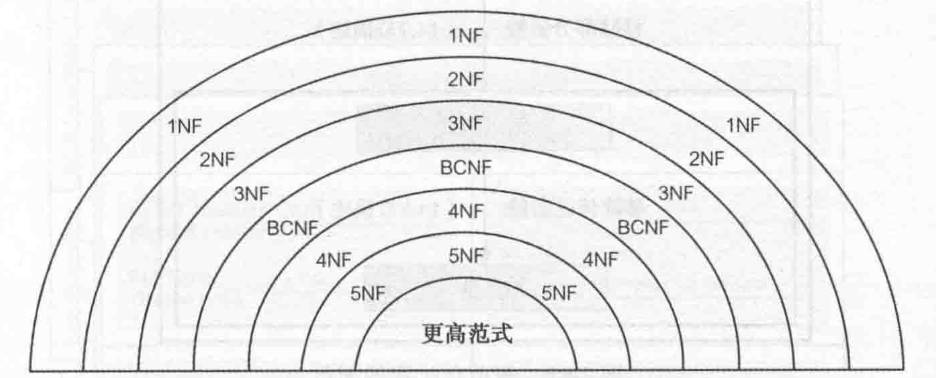# 数据库系统（8）：规范化

规范化 ( $Normalization$ ) 是一种数据库设计技术，从分析属性之间的联系入手。规范化使用一系列测试，描述为范式 ( $normal\ \ forms$ ) 帮助我们确定这些属性的最佳组合，最终生成一组适当关系。

## 1. 目的

规范化的目的是确定一组合适的关系以支持企业的数据需求。该关系具有以下性质：

• 属性的个数最少，且是必需的
• 具有紧密逻辑联系，描述为函数依赖 ( $functional\ \ dependency$ ) 的所有属性均在一个关系中
• 最少的冗余，即每个属性仅出现一次，除了作为外键的属性，因为连接相关关系必须用到外键

具有一组合适的关系，数据库会易于用户访问，数据易于维护，在计算机上也会占有较小的存储空间。

## 2. 支持

规范化有两种使用方法。方法 $1$ 将规范化视为一种自下而上 ( $bottom-up$ ) 的独立的数据库设计技术。方法 $2$ 将规范化作为一种确认技术使用，即用规范化检验关系的结构，而这些关系的建立可能采用自上而下的方法，如ER建模等。

## 3. 数据冗余与更新异常

关系数据库设计的一个目的就是将属性组合成关系时保证最少的数据冗余。当然，关系数据库的运行也依赖于一定的数据冗余的存在，一般是以主键或者候选键的多个副本的形式出现，作为外键表示数据间联系。存在冗余数据的关系可能存在一些问题，如更新异常 ( $update\ \ anomalies$ )。更新异常又可分为插入异常、删除异常和修改异常。$Staff$ 和 $Branch$ 是不存在数据冗余的两个表。$StaffBranch$ 是将上两个表合起来的存在数据冗余的表，同一个分公司的信息会重复出现。

### 3.1 插入异常

插入异常主要有两类：

• 在向 $StaffBranch$ 中插入新员工时，若同一家分公司信息不同，则会产生一致性问题；
• 在向 $StaffBranch$ 中插入新的分公司时，由于没有员工，因此员工信息都应设为 $null$ 。但是主键 $staffNo$ 不能为空，否则会违反实体完整性约束。

### 3.2 删除异常

从 $StaffBranch$ 中删除元组时，若该元组中的员工信息是某个分公司的最后的员工，则该分公司的信息也会被删除。

### 3.3 修改异常

当要修改 $StaffBranch$ 中某个分公司的属性值时，必须依次修改所有包含该分公司信息的元组。

上述的所有问题都可以通过将 $StaffBranch$ 分为 $Staff$ 和 $Branch$ 来解决，即把较大的关系分为较小的关系。这时有两个重要特性：

• 无损连接 ( $lossless-join$ ) ：该特性确保了原关系的任意实例信息能够通过较小关系的对应实例确定；
• 依赖保持 ( $dependency\ \ preservation$ ) ：该特性确保了只需简单地在较小的关系上支持约束，就可以继续支持原关系的约束。即不需要连接操作就可以判断是否符合原关系的约束。

## 4. 函数依赖

函数依赖描述了属性之间的联系。

### 4.1 特征

假设有一关系模式，具有属性 ($A$, $B$, $C$,…, $Z$) ，我们使用全域关系 ( $universal\ \ relation$ ) $R$ = ( $A$, $B$, $C$,…, $Z$ ) 来描述数据库。函数依赖描述一个关系中属性之间的联系，若 $A$ 的每个值都和 $B$ 中的一个唯一的值相对应，则称 $B$ 函数依赖于 $A$ ，记为 $A \rightarrow B$ ( $A$, $B$ 可能由一个或者多个属性组成 )。
函数依赖是属性在关系中的一种语义特性，表明属性和属性之间是如何关联起来的。当存在某一函数依赖时，这个依赖就被视为属性之间的一种约束。当 $B$ 函数依赖于 $A$ 时，对于 $A$ 的每一个值，都有唯一的 $B$ 的值与之对应。因此当两个元组 $A$ 的值相等时，它们 $B$ 的值也一定相等。但是反过来，当它们 $B$ 的值相等时，$A$ 的值却不一定相等，因为不同的 $A$ 的值可以对应同一个 $B$ 的值。因此也可以把 $B$ 函数依赖于 $A$ 称为 $A$ 函数决定 $B$ ，$A$ 称为决定方 ( $determinant$ )。在确定一个关系中属性之间的函数依赖时，必须明确它是仅当属性取某一特定值时成立还是属性取集合中任意值时成立，即函数依赖是关系模式的性质还是某个实例的性质，如果是前者，称为恒成立 ( $holds\ \ for\ \ all\ \ time$ ) 函数依赖 。当决定方具有的属性是保证右边属性的函数依赖于它所必不可少的属性时，我们称之为完全函数依赖 ( $full\ \ functional\ \ dependency$ )。否则，称为部分函数依赖。
规范化时要用到的函数依赖具有下列性质：

• 函数依赖左边的属性与右边属性是一对一的联系（反过来可以是一对一，也可以是一对多）；
• 恒成立
• 完全函数依赖

此外，还有一种函数依赖称为传递依赖 ( $transitive\ \ dependency$ )。若 $A \rightarrow B$，$B \rightarrow C$ ，则 $C$ 通过 $B$ 传递依赖于 $A$ （假设 $A$ 并不函数依赖于 $B$ 或 $C$ ）。若关系中存在传递依赖，可能引起更新异常。

### 4.2 识别

$A$ $B$ $C$ $D$ $E$
$a$ $b$ $z$ $w$ $q$
$e$ $b$ $r$ $w$ $p$
$a$ $d$ $z$ $w$ $t$
$e$ $d$ $r$ $w$ $q$
$a$ $f$ $z$ $s$ $t$
$e$ $f$ $r$ $s$ $t$
1. 当 $A$ 为 $a$ 时，$C$ 就为 $z$ ；当 $A$ 为 $e$ 时，$C$ 就为 $r$ 。因此 $A \rightarrow C$ ，也可以说 $C \rightarrow A$ ；
2. 同理，$B \rightarrow D$ ， 但是不能说 $D \rightarrow B$ ，因为当 $D$ 为 $w$ 时对应多个 $B$ 的值，即 $D$ 与 $B$ 之间是一对多的联系；
3. $E$ 与其他属性的变化都不一致，因此不能函数决定其他属性。但是当 $A$ 和 $B$ 的取值固定时，$E$ 的取值也固定，因此可以说 $A, B \rightarrow E$ 。同理 $B, C \rightarrow E$ 。

### 4.3 利用函数依赖确定主键

确定函数依赖的主要目的时确定该关系必须满足的完整性约束集。首先要考虑的就是候选键。上述的关系的决定方有 $A$, $B$, $C$, $(A, B)$, $(B, C)$ 。综合来看，只有 $(A, B)$ 和 $(B, C)$ 能够决定其他所有属性，因此它们都是候选键。由于长度一样，因此都可以作为主键。

## 5. 过程

规范化的过程包括一系列步骤，每一步都对应着某种具有已知性质的特定范式。最早提出的三个范式为第一范式 ( $1NF$ ) 、第二范式 ( $2NF$ ) 和第三范式 ( $3NF$ ) ，后来又提出了一种增强的第三范式，称为 $Boyce-Codd$ 范式 ( $BCNF$ ) 。也存在更高层的第四范式 ( $4NF$ ) 和第五范式 ( $5NF$ ) ，但是需要用到的情况相当少。除了第一范式外，这些范式都是基于关系的属性间函数依赖的。对于关系数据模型，在建立关系时只有满足 $1NF$ 的需求是必须的，后面其他范式都是可选的。但是为了避免更新异常，通常建议将规范化至少进行到 $3NF$ 。非范式 ( $Unnormalized\ \ Form$, $UNF$ ) 是包含一个或者多个重复组的表。非范式的表格称为非规范化的表 ( $unnormalized\ \ table$ ) 。在使用范式之前，我们需要将需求先转化为非范式表的形式，然后将其逐渐分解直至满足每一种范式的要求。规范化过程通过使用一系列的关系代数投影对原始关系进行分解，这是一种无损连接 ( $lossless-join$ ) 分解，也称为无损耗 ( $nonloss-$ ) /无附加 ( $nonadditive-$ ) 连接分解，即反向使用自然连接操作就可以得到原关系。

## 6. 第一范式

属于第一范式的关系的每一行和每一列相交的位置有且仅有一个值。为了将非规范化的表转化为第一范式，我们需要确定并删除表中的重复组。重复组可以是一个属性或者一组属性，包含表中对应关键属性的一个实例时可能出现多个值的属性。通常有两种方法：

1. 在含有重复数据的那些行的空白列上输入合适的数据。这种方法通常被看作是对表的平板化 ( $flattening$ ) 处理；
2. 将重复数据单独移到一个新的关系中，同时也将原来关系中的关键属性复制到这个关系中。

这两种方法都是可行的。但是第一种方法引入了较多的冗余，可能会导致更新异常；第二种方法则创建了更多的关系，降低了冗余，但是需要更多工作。

## 7. 第二范式

第二范式是满足第一范式的要求并且每个非主键属性都完全函数依赖于主键的关系。第二范式基于完全函数依赖的概念，使用于具有合成键的关系，即主键由两个或两个以上的属性组成。主键仅包含一个属性的关系已经至少是 $2NF$ 的。如果存在部分依赖，就要将部分依赖的属性从原关系移到一个新的关系中去，包括这些属性的决定方。
将 $1NF$ 转化为 $2NF$ ，需要先找出所有的函数依赖，利用这些函数依赖来确定主键。再通过确定主键上是否存在部分依赖来验证该关系是否是 $2NF$ 。如果非 $2NF$ ，则要创建新关系，将存在部份依赖的非主键属性移至其中。

## 8. 第三范式

尽管 $2NF$ 的关系比 $1NF$ 关系的数据冗余度低，但是仍然存在更新异常的问题，此时的更新异常是由传递依赖引起的。第三范式满足第一范式和第二范式的要求并且所有非主键属性都不传递依赖于主键的关系，即消除 $2NF$ 中的传递依赖。
如果关系中的所有非主键属性都完全函数依赖于它们的主键，则它们已经是 $3NF$ 。而如果除了主键之外还传递依赖于其他属性，那么就要通过分解关系来消除该依赖。

## 9. 一般化定义

在对 $2NF$ 和 $3NF$ 更一般化的定义中，我们规定：属于任何一个候选键的属性都叫做主属性 ( $candidate-key\ \ attribute$ )；提到部份依赖、完全依赖和传递依赖时不仅仅是基于主键，而是基于所有候选键。

• 第二范式是满足第一范式的要求并且每个非主属性都完全函数依赖于任何一个候选键的关系。
• 第三范式是满足第一范式和第二范式的要求并且没有一个非主属性传递依赖于任何一个候选键的关系。

## 10. BCNF

$Boyce-Codd$ 范式是每个函数依赖的决定方都是候选键的关系。$BCNF$ 与 $3NF$ 的区别表现在对于一个函数依赖 $A \rightarrow B$ ，$3NF$ 允许 $B$ 是主键属性而 $A$ 非候选键。一般情况下 $3NF$ 都是 $BCNF$ ，但是在一些特殊性情况下会违反，这些情况有：

• 关系中包含两个（或更多个）合成候选键
• 候选键互相重叠，通常至少都包含一个相同属性
$clientNo$ $interviewDate$ $interviewTime$ $staffNo$ $roomNo$
$CR76$ $13-May-14$ $10.30$ $SG5$ $G101$
$CR56$ $13-May-14$ $12.00$ $SG5$ $G101$
$CR74$ $13-May-14$ $12.00$ $SG37$ $G102$
$CR56$ $1-Jul-14$ $10.30$ $SG5$ $G102$

关系 $ClientInterview$ 中有三个候选键 ( $clientNo$, $interviewDate$ ), ( $staffNo$, $interviewDate$, $interviewTime$ ), ( $roomNo$, $interviewDate$, $interviewTime$ ) ，选择 ( $clientNo$, $interviewDate$ ) 作为主键，则函数依赖如下所示：

\begin{aligned} &fd1\quad clientNo, interviewDate \rightarrow interviewTime, staffNo, roomNo\quad\text{(主键)}\\ &fd2\quad staffNo, interviewDate, interviewTime \rightarrow clientNo\quad\text{(候选键)}\\ &fd3\quad roomNo, interviewDate, interviewTime \rightarrow staffNo, clientNo\quad\text{(候选键)}\\ &fd4\quad staffNo, interviewDate \rightarrow roomNo\quad \end{aligned}

$fd1$, $fd2$, $fd3$ 都是候选键，而 $fd4$ 不是。因此这个关系是 $3NF$ ，而非 $BCNF$ 。在非 $BCNF$ 的关系中可能会出现更新异常。例如当我们要更新 $interviewDate$ 为 $13-May-14$ ，$staffNo$ 为 $SG5$ 的行的 $roomNo$ 属性时，要同时更新两行，否则就会导致不一致问题。
将 $3NF$ 转化为 $BCNF$ ，必须消除不满足 $BCNF$ 的函数依赖。为此，我们可以分解关系，如下：

$clientNo$ $interviewDate$ $interviewTime$ $staffNo$
$CR76$ $13-May-14$ $10.30$ $SG5$
$CR56$ $13-May-14$ $12.00$ $SG5$
$CR74$ $13-May-14$ $12.00$ $SG37$
$CR56$ $1-Jul-14$ $10.30$ $SG5$

$staffNo$ $interviewDate$ $roomNo$
$SG5$ $13-May-14$ $G101$
$SG37$ $13-May-14$ $G102$
$SG5$ $1-Jul-14$ $G102$

将 $3NF$ 通过关系分解的形式转化为 $BCNF$ 的过程中会丢失某个函数依赖，在上例中是 $fd3$ 。如果这并非我们想要的，那么只需要将规范化进行到 $3NF$ 即可。是否将关系规范化到 $BCNF$ ，取决于是 $fd4$ 的数据冗余带来的影响更大还是 $fd3$ 丢失带来的影响更大。